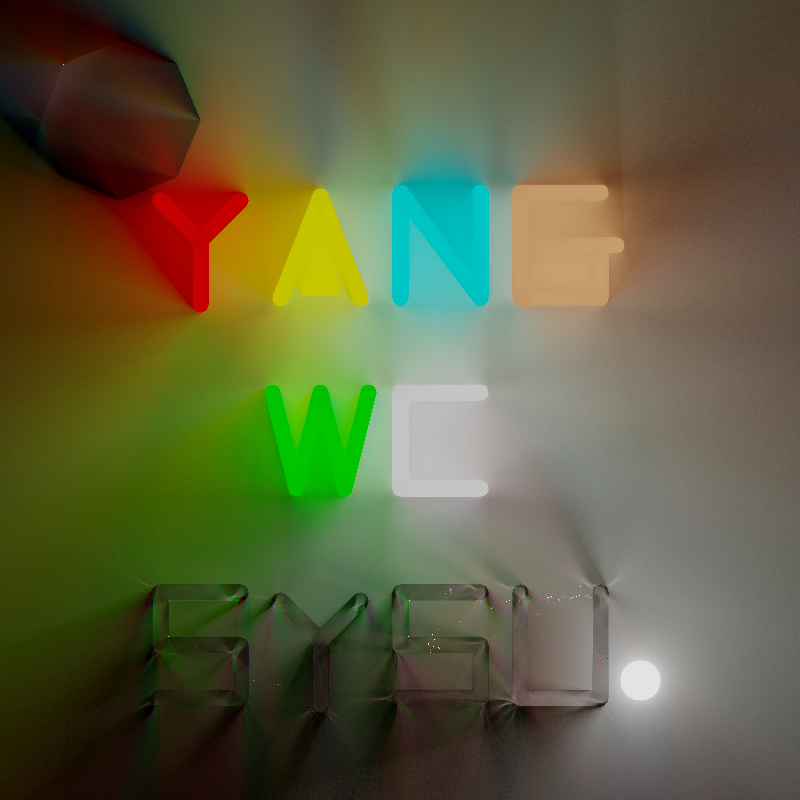# 二维渲染2D Rendering：2D Lighting• 二维渲染
• SDF构造实体几何
• 比尔-郎伯定律
• 实现效果
• 参考资料
2D Lighting
偶然在知乎上看到图形学大佬的几篇关于二维渲染绘制的文章，感觉非常有趣，二维的相对而言比较简单但是涉及到的知识也不少，遂记录下来一些自己的学习总结。一些内容跟前面光线追踪的重合了，所以就不细细展开了。本文主要是关于SDF方面的内容。 ## 一、二维渲染   我们要绘制的世界是一个二维的场景，场景中有发光的二维体。对于一个二维屏幕上的像素，我们要求在360度方向上接收的光照值，故给定二维空间的坐标$(x,y)$，渲染方程为对$[0,2\pi]$方向的单重积分： $$L_o(x,y)=\int_0^{2\pi}L_i(x,y,\theta)d\theta \tag {1}$$   在这里我们就对二维图像的每个像素求解方程$(1)$的积分公式，用tbb多线程库加速渲染，然后将像素矩阵用stb_image保存成png文件。原点在图像的左上角。   由于被积函数$L(x,y,\theta)$并没有一个显示的数学表达式，我们无法求解公式$(1)$单重积分的解析解，因此采用蒙特卡洛数值积分法。我们首先考虑随机均匀采样$N$个方向，每个方向用$\theta_1,\theta_2,...,\theta_N$表示，因为是均匀采样，所以概率密度函数$pdf=\frac {1}{2\pi}$，蒙特卡洛数值积分公式如下： $$L_o(x,y)=\frac 1N\Sigma_{i=1}^N\frac{L_i(x,y,\theta)}{\frac{1}{2\pi}} =\frac {2\pi}N\Sigma_{i=1}^N{L_i(x,y,\theta)} \tag {2}$$   因此一个随机均匀采样的蒙特卡洛积分如下所示，此外在这里我们不考虑实际单位，所以实现时把系数$2\pi$去掉了。   紧接着我们将实现trace函数，这个函数返回给定方向上的光照值。我们采用光线步进法（Ray Marching），场景中的物体以符号距离场（Signed Distance Field，简称SDF）表示，符号距离场是一个这样的映射：$\phi:R^2\to R$，具有如下的性质：   (1).当$\phi(x)>0$时，坐标$x$位于场景的物体外面，$x$到最近物体表面的距离为$\phi(x)$；   (2).当$\phi(x)<0$时，坐标$x$位于场景的物体里面，$x$到最近物体表面的距离为$-\phi(x)$；   (3).当$\phi(x)="0$时，坐标$x$恰好位于场景的物体表面上。"   一个圆心为$c$、半径为$r$的圆盘sdf为：  \phi_{disk}(x)="||x-c||-r" \tag {3}